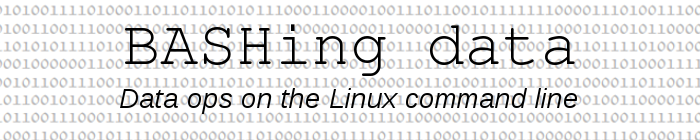For a full list of BASHing data blog posts see the index page.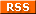# Building a molar mass calculator

You can find a lot of chemical-formula parsers online, notably in Python and Java. They usually aim to do something fairly simple: given a formula like "CaCl2" or "C6H12O6", produce mappings like this:

Ca   1
Cl   2

C   6
H   12
O   6

Being an AWK fanatic enthusiast, I wondered how hard that would be to do with pure AWK and whether I could output the compound's molar mass (g/mol; also known as molecular weight).

It took some tinkering, but the script "moma" (see end of post) does the job nicely for formulas like "CaCl2" and "(CH3)2CHCOOH". It doesn't work for nested groups as in "TiCl2[(CH3)2PCH2CH2P(CH3)2]2", or for hydrates formatted like "CuSO4.5H20"; these would need to be converted to "TiCl2(CH3)4P2(CH2)2(CH2)2P2(CH3)4" and "CuSO4(H20)5".

Getting the atomic weights. I first built a space-separated table of element symbols and atomic weights ("atwts") from an online reference here. I put the table in my ~/scripts/ folder: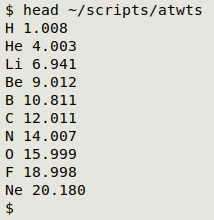Building a test formula. To make things difficult for myself I put together bits and pieces to make a completely fictitious and improbable compound, then stored the formula in the shell variable "chimera" so I wouldn't have to type it repeatedly:

chimera="(C2H3)3CrFrCNFe2Al3P4S5(OH)3(SO4)6Bi12"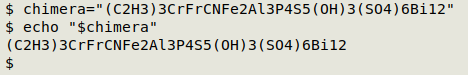Dividing the formula into grouped and ungrouped. The processing of grouped and ungrouped strings will be different, so I need to split the formula. A simple way to do this is with AWK's field specifiers. I use the regex [(][A-Za-z0-9]+[)][0-9]+ to define a group: 1 or more letters and numbers inside parentheses, followed by 1 or more numbers. If I specify this regex as the field pattern with FPAT, then loop through the fields in the formula and print the fields, I get a list of the groups:

awk -v FPAT="[(][A-Za-z0-9]+[)][0-9]+" \
'{for (i=1;i<=NF;i++) print \$i}' <<<"\$chimera"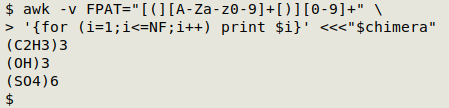Conversely, I can let that regex be the field separator (FS), loop through the fields and printf the fields as a concatenated string:

awk -v FS="[(][A-Za-z0-9]+[)][0-9]+" \
'{for (i=1;i<=NF;i++) printf("%s",\$i)}' <<<"\$chimera"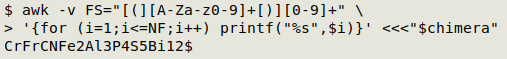Processing the groups - part 1. Each group starts with a "(", and finishes with ")" and a number. If I specify those parentheses as field separators, then the bits inside the parentheses will be field 2 and the the trailing number will be field 3. I can "multiply" each group with field 3 to build a list. Each item on the list will be an "ungrouped" formula, so I'll leave the group processing for a moment to work on ungrouped formulas.

awk -v FPAT="[(][A-Za-z0-9]+[)][0-9]+" \
'{for (i=1;i<=NF;i++) print \$i}' <<<"\$chimera" \
| awk -F"[()]" '{for (i=1;i<=\$3;i++) printf("%s\n",\$2)}'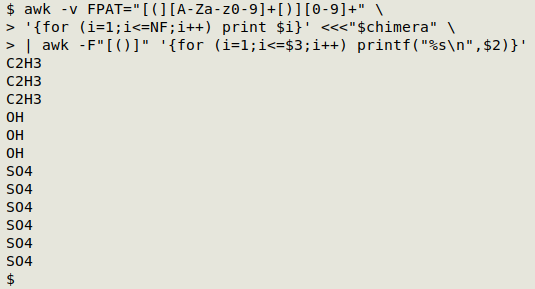Processing the ungrouped strings. This step uses a fairly complicated but logical AWK command. It first defines a field as either an uppercase letter followed by a lowercase letter followed by zero or more numbers ("Ni" or "Fe2"), or as an uppercase letter followed by zero or more numbers ("N" or "P4"). Each of the fields is then checked to see it contains a number. If it does, the field is split using the number as delimiter. The split-out symbols are stored in an array "a" and the delimiting numbers in another array, "sep". AWK then prints the first element each in "a" and "sep" with the default separator, a space. If the field doesn't contain a number, AWK prints the field, a space and the number "1":

awk -v FS="[(][A-Za-z0-9]+[)][0-9]+" \
'{for (i=1;i<=NF;i++) printf("%s",\$i)}' <<<"\$chimera" \
| awk -v FPAT="[A-Z][a-z][0-9]*|[A-Z][0-9]*" \
'{for (i=1;i<=NF;i++) \
if (\$i ~ /[0-9]/) {split(\$i,a,/[0-9]+/,sep); \
print a,sep} \
else print \$i,"1"}'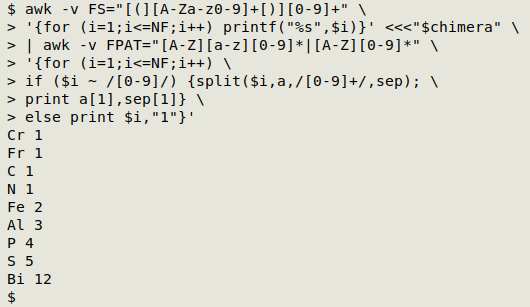Processing the groups - part 2. Now that I have a way to process ungrouped strings, I can pass the part-processed groups to that last AWK command to finish their processing. Here I've piped the output to column to save space in the screenshot:

awk -v FPAT="[(][A-Za-z0-9]+[)][0-9]+" \
'{for (i=1;i<=NF;i++) print \$i}' <<<"\$chimera" \
| awk -F"[()]" '{for (i=1;i<=\$3;i++) printf("%s\n",\$2)}' \
| awk -v FPAT="[A-Z][a-z][0-9]*|[A-Z][0-9]*" \
'{for (i=1;i<=NF;i++) \
if (\$i ~ /[0-9]/) {split(\$i,a,/[0-9]+/,sep); \
print a,sep} \
else print \$i,"1"}'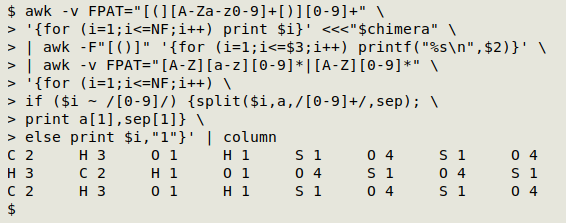Store outputs in variables. For clarity I've put the grouped and ungrouped outputs into the variables "group" and "ungroup":

group=\$(awk -v FPAT="[(][A-Za-z0-9]+[)][0-9]+" \
'{for (i=1;i<=NF;i++) print \$i}' <<<"\$chimera" \
| awk -F"[()]" '{for (i=1;i<=\$3;i++) printf("%s\n",\$2)}' \
| awk -v FPAT="[A-Z][a-z][0-9]*|[A-Z][0-9]*" \
'{for (i=1;i<=NF;i++) \
if (\$i ~ /[0-9]/) {split(\$i,a,/[0-9]+/,sep); \
print a,sep} \
else print \$i,"1"}')

ungroup=\$(awk -v FS="[(][A-Za-z0-9]+[)][0-9]+" \
'{for (i=1;i<=NF;i++) printf("%s",\$i)}' <<<"\$chimera" \
| awk -v FPAT="[A-Z][a-z][0-9]*|[A-Z][0-9]*" \
'{for (i=1;i<=NF;i++) \
if (\$i ~ /[0-9]/) {split(\$i,a,/[0-9]+/,sep); \
print a,sep} \
else print \$i,"1"}')

Calculate masses. I now have two space-separated lists of symbols and corresponding numbers. To get the total mass I first put the "atwts" table into an array where the index string is the symbol and the value string is the atomic weight. I then process "group" and "ungroup", line by line. When a symbol is in the array, AWK adds to the variable "sum" the product of the atomic weight and the number corresponding to that symbol. In an END statement after both "group" and "ungroup" are processed, AWK prints the aggregated total mass for the formula:

awk 'FNR==NR {a[\$1]=\$2; next} \
\$1 in a {sum+=\$2*a[\$1]} \
END {print sum" g/mol"}' \
~/scripts/atwts <(echo "\$group") <(echo "\$ungroup")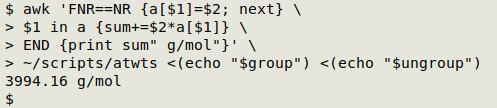And yes, 3994.16 is the molar mass of (C2H3)3CrFrCNFe2Al3P4S5(OH)3(SO4)6Bi12!

Demos of "moma" below. All molar masses are correctly calculated from the "atwts" table.

Please note, however, that the script doesn't validate formulas, and will return a number even if the formula contains a mistake. For example, "Ca3(PO4(2" returns "215.204 g/mol", not the correct 310.174 g/mol for Ca3(PO4)2. The way I've used "moma" is to paste in valid formulas that I've copied from documents and Web sources.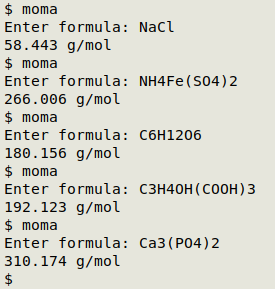The moma script:

#!/bin/bash

read -p "Enter formula: " foo

group=\$(awk -v FPAT="[(][A-Za-z0-9]+[)][0-9]+" \
'{for (i=1;i<=NF;i++) print \$i}' <<<"\$foo" \
| awk -F"[()]" '{for (i=1;i<=\$3;i++) printf("%s\n",\$2)}' \
| awk -v FPAT="[A-Z][a-z][0-9]*|[A-Z][0-9]*" \
'{for (i=1;i<=NF;i++) \
if (\$i ~ /[0-9]/) {split(\$i,a,/[0-9]+/,sep); \
print a,sep} \
else print \$i,"1"}')

ungroup=\$(awk -v FS="[(][A-Za-z0-9]+[)][0-9]+" \
'{for (i=1;i<=NF;i++) printf("%s",\$i)}' <<<"\$foo" \
| awk -v FPAT="[A-Z][a-z][0-9]*|[A-Z][0-9]*" \
'{for (i=1;i<=NF;i++) \
if (\$i ~ /[0-9]/) {split(\$i,a,/[0-9]+/,sep); \
print a,sep} \
else print \$i,"1"}')

awk 'FNR==NR {a[\$1]=\$2; next} \
\$1 in a {sum+=\$2*a[\$1]} \
END {print sum" g/mol"}' \
~/scripts/atwts <(echo "\$group") <(echo "\$ungroup")

exit

Last update: 2021-03-24
The blog posts on this website are licensed under a